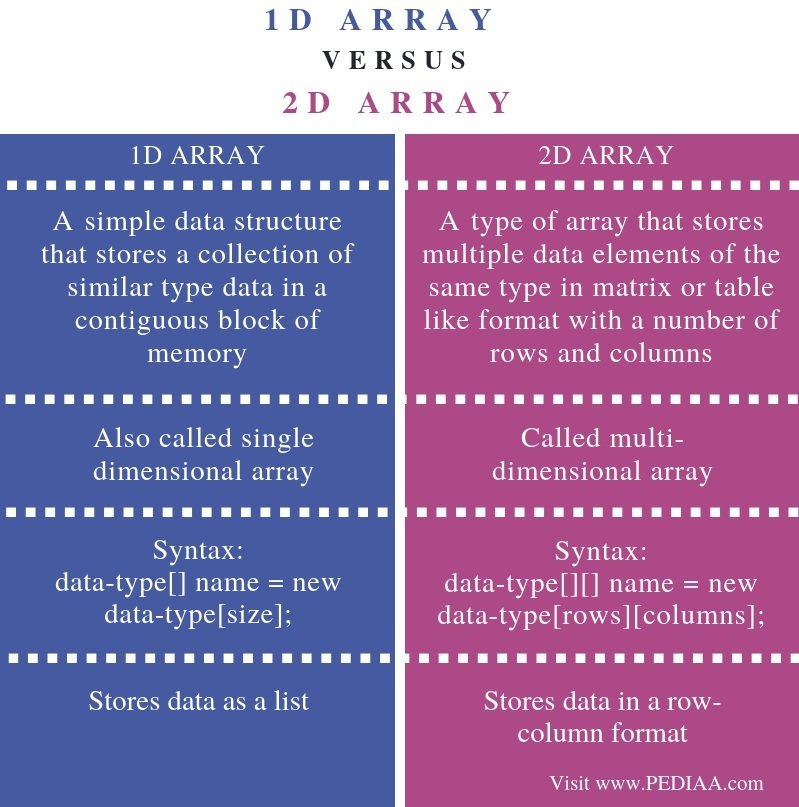# What is the Difference Between 1D and 2D Array

The main difference between 1D and 2D array is that the 1D array represents multiple data items as a list while 2D array represents multiple data items as a table consisting of rows and columns.

A variable is a memory location to store data of a specific type. Sometimes, it is necessary to store a set of items of the same data type. An array allows storing multiple items of the same data type. The elements in the array are in subsequent memory locations. There are two types of arrays as one dimensional (1D) array and two dimensional (multi-dimensional) arrays.

### Key Areas Covered

1. What is 1D Array
– Definition, Functionality
2. What is 2D Array
– Definition, Functionality
3. What is the Difference Between 1D and 2D Array
– Comparison of Key Differences

### Key Terms

1D Array, 2D Array, Array, Multi-Dimensional Array, Single Dimensional Array## What is 1D Array

1D array or single dimensional array stores a list of variables of the same data type. It is possible to access each variable using the index.In Java language, int[] numbers; declares an array called numbers. Then, we can allocate memory for that array using ‘new’ keyword as follows.

numbers= new int;

This array is capable of storing 10 integer values.

We can combine the above two statements together and write as follows.

int numbers = new int;

Below is an example of assigning values to the array.

numbers ={1,2,3,4,5,6,7,8,9,10};

The starting index of an array is 0. Therefore, the element in the 0th index is 1. The element in the 1st index is 2. The element in the 2nd index is 3, etc. The index of the final element is 9.

If the programmer wants to store number 50 on the 2nd index, he can write the statement as follows.

numbers = 50;

## What is 2D Array

2D array or multi-dimensional array stores data in a format consisting of rows and columns.For example, int[][] numbers; declares a 2D arrays.

numbers = new int ;

The above statement allocates memory for a 2D array of 2 rows and 3 columns.

We can combine the above two statements together and write the statement as follows.

int[][] numbers = new int;

Below is an example of assigning values to the 2D array.

int[][] numbers = { {10,20,30}, {50,60,70}};

Similar to a 1D array, the starting index of the 2D array is also 0. This array has two rows and three columns. The indexes of the rows are 0 and 1 while the indexes of columns are 0, 1 and 2. The element 10 is in the 0th row 0th column position. Number 20 is in the 0th row, 1st column position. Number 70 is in 1st row, 2nd column position.

numbers = 50;

Above statement assigns number 50 to 1st row, 2nd column position.

## Difference Between 1D and 2D Array

### Definition

A 1D array is a simple data structure that stores a collection of similar type data in a contiguous block of memory while the 2D array is a type of array that stores multiple data elements of the same type in matrix or table like format with a number of rows and columns. Thus, this is the main difference between 1D and 2D array.

### Synonyms

A 1D array is also called single dimensional array while the 2D array is called multi-dimensional array.

### Declaration

Declaration is another difference between 1D and 2D array. The syntax for 1D array is, data-type[] name = new data-type[size]; while the syntax for 2D array is, data-type[][] name = new data-type[rows][columns];

### Storing Data

Moreover, a major difference between 1D and 2D array is that 1D array stores data as a list while 2D array stores data in a row-column format.

### Conclusion

There are two types of arrays as 1D and 2D arrays. The main difference between 1D and 2D array is that the 1D array represents multiple data items as a list while 2D array represents multiple data items as a table consisting of rows and columns.

##### Reference:

1. Krishna, Appili Vamsi. “Arrays 1D and 2D, and Multi-Dimensional.” LinkedIn SlideShare, 27 Mar. 2017, Available here.
2. “Java 1D Array.” HackerRank, Available here.
3. UC Berkeley, “Dimensional Arrays”,  Available here, Available here.

##### Image Courtesy:

1. “CPT-programming-array” By Pluke – Own work (CC0) via Commons Wikimedia
2. “Array2” By  Jarkko Piiroinen assumed  – No machine-readable source provided. Own work assumed (based on copyright claims) (Public Domain) via Commons Wikimedia